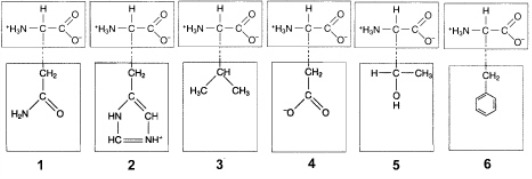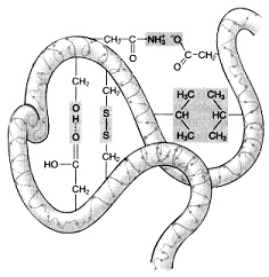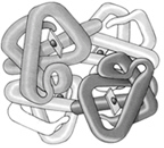• FAQ
• Contact/
/
/
41. If tyrosine and isoleucine undergo condensation, the new bond that
Not my Question
Flag Content

# Question : 41. If tyrosine and isoleucine undergo condensation, the new bond that : 1856085

41. If tyrosine and isoleucine undergo condensation, the new bond that is formed is between the:
A. oxygen of the R group and the hydrogen of the amino group.
B. carbon of the R group and the nitrogen of the amino group.
C. carbon of the carboxyl group and the hydrogen of the R group.
D. carbon of the carboxyl group and the hydrogen of the amino group.
E. carbon of the carboxyl group and the nitrogen of the amino group.

42. Figure 3-2
Use the figure below to answer the corresponding question(s).In Figure 3-2, ionic bonds would form between the R groups of which amino acids?
A. 1 and 3
B. 2 and 4
C. 3 and 5
D. 4 and 6
E. 3 and 6

43. Figure 3-2
Use the figure below to answer the corresponding question(s).Hydrophobic interactions would occur between the R groups of which two amino acids in Figure 3-2?
A. 1 and 4
B. 2 and 5
C. 3 and 6
D. 2 and 4
E. 3 and 5

44. The tertiary structure of proteins is typified by the:
A. association of several polypeptide chains by weak bonds.
B. order in which amino acids are joined in a peptide chain.
C. bonding of two amino acids to form a dipeptide.
D. folding of a peptide chain to form an alpha helix.
E. three-dimensional shape of an individual polypeptide chain.

45. All of the following types of chemical bonds are responsible for maintaining the tertiary structure of this polypeptide except:A. ionic bonds.
B. hydrogen bonds.
C. hydrophobic interactions.
D. disulfide bonds.
E. peptide bonds.

46. This functional group forms bridges that help stabilize protein quaternary structure:
A. hydroxyl
B. carbonyl
C. amino
D. phosphate
E. sulfhydryl

47. Molecular chaperones are proteins that mediate the folding process of:
A. other proteins.
B. amino acids.
C. lipids.
D. DNA.
E. sugars.

48. If the differently shaded portions of this molecule represent different polypeptide chains, then this figure is representative of:A. an amino acid.
B. the quaternary structure of a protein.
C. a steroid hormone.
D. cellulose.
E. a carotenoid.

49. Which of the following statements is true of proteins?
A. Proteins lose some or all of their normal activity if their three-dimensional structure is disrupted.
B. Proteins are composed of ribose, phosphate, and a nitrogen-containing base.
C. The activity of proteins is independent of temperature and pH.
D. Denaturation is usually reversible.
E. All proteins are enzymes.

50. This molecule transmits heredity information:
A. cholesterol.